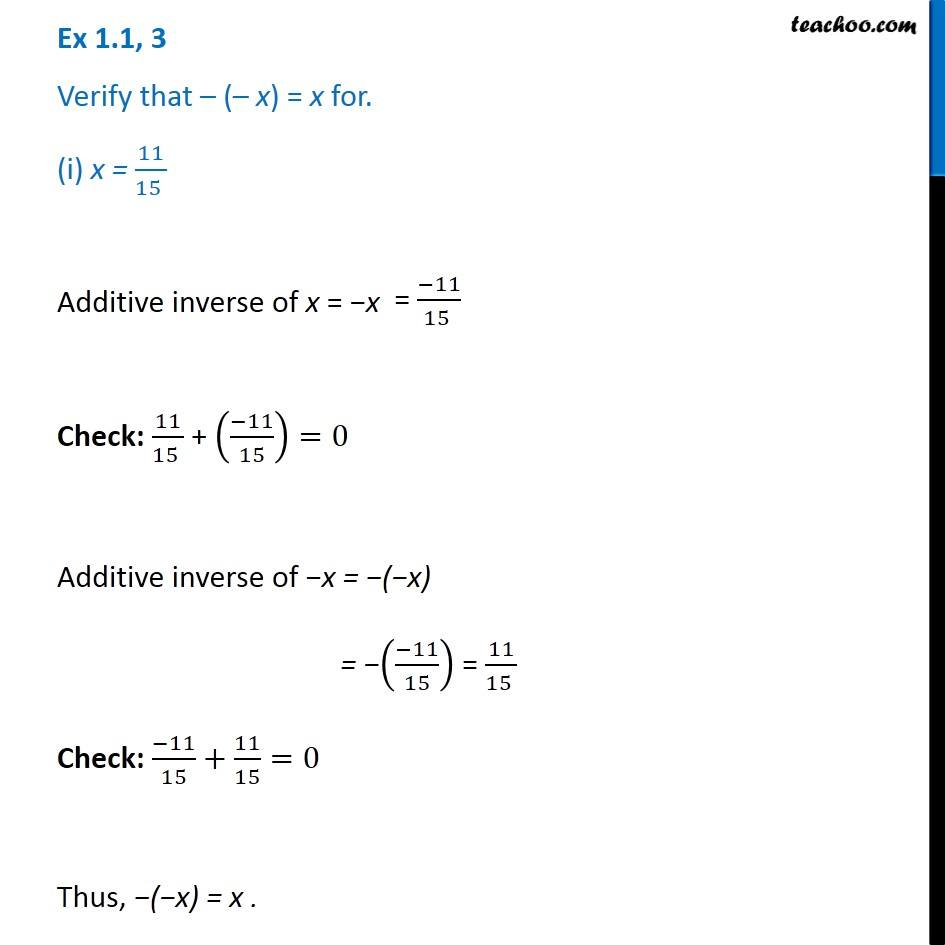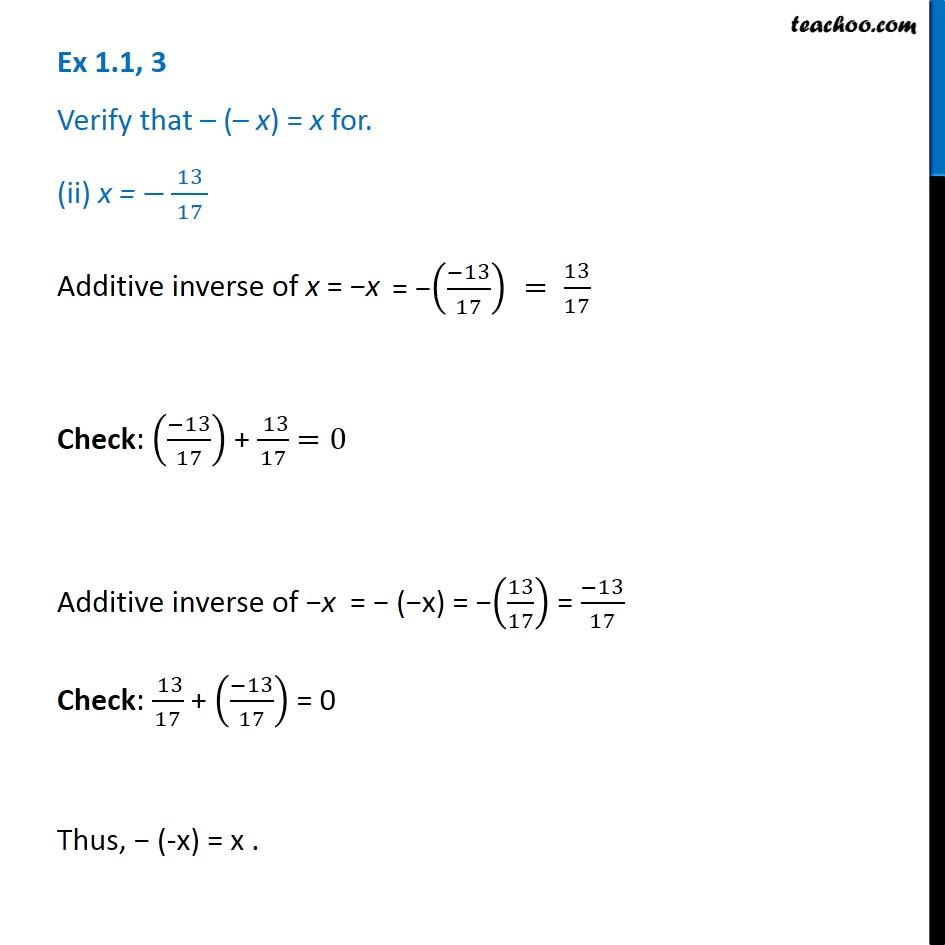Ex 1.1

Chapter 1 Class 8 Rational Numbers
Serial order wiseGet live Maths 1-on-1 Classs - Class 6 to 12

### Transcript

Ex 1.1, 3 Verify that ( x) = x for. (i) x = 11/(15 ) Additive inverse of x = x Check: 11/(15 ) + (( 11)/15)=0 Additive inverse of x = ( x) = (( 11)/15) = 11/(15 ) Check: ( 11)/15+11/15=0 Thus, ( x) = x . Ex 1.1, 3 Verify that ( x) = x for. (ii) x = 13/( 17 ) Additive inverse of x = x Check: (( 13)/17) + ( 13)/17 =0 Additive inverse of x = ( x) = (13/17) = ( 13)/17 Check: ( 13)/17 + (( 13)/17) = 0 Thus, (-x) = x .